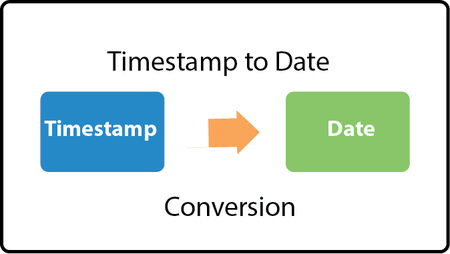# How to Convert Unix Timestamp to Datetime in Python

Often timestamp information is stored in Unix timestamp format in databases and applications. Unix timestamp format is the number of milliseconds from epoch (Jan 1, 1970). But you may need to convert this information into datetime values in python to display human readable values on your websites and apps. In this article, we will learn how to convert Unix timestamp to datetime Python.

## How to Convert Unix Timestamp to Datetime in Python

Here is an example of Unix Timestamp.

```from datetime import datetime
ts = int('1234567890')
```

Here are some of the different ways to convert this into datetime in Python.

`datetime.utcfromtimestamp(ts).strftime('%Y-%m-%d %H:%M:%S')`

Please note, the above command will display time as per local timezone. If you do not want it then you can use UTC timezone format.

`datetime.datetime.utcfromtimestamp(posix_time).strftime('%Y-%m-%dT%H:%M:%SZ')`

In the above command, we first convert Unix timestamp to datetime object using utcfromtimestamp() function. Then we convert it into a string of our desired format using strftime(). You can change the date format as per your requirement.

If you get an error saying ‘year is out of range’, then probably the timestamp is in milliseconds and needs to be divided by 1000 to convert into seconds.

`datetime.utcfromtimestamp(ts/1000).strftime('%Y-%m-%d %H:%M:%S')`

In this article, we have learnt how to convert Unix timestamp to Datetime.